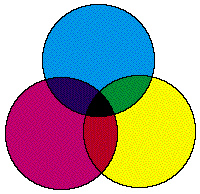## Question 1

1A. (5 points) Describe the physics (in terms of changes to various spectra) when two colored light sources are combined.  What's the difference between combining light sources and combining two colored filters?

A colored light source can be represented as a spectral energy distribution, a graph of how much energy the light source puts out at each wavelength.  When we combine two light sources, we are combining their energies.  To find the spectral energy distribution of the result, we sum the energies of the two sources at each wavelength.  Since we are adding light energies, we call this additive color mixing.

A colored filter can also be thought of in terms of a spectral energy distribution, but this time in terms of how much energy at each wavelength the filter blocks.  When we combine two filters, we combine their blocking powers multiplicatively: if filter A passes 40% of the energy at a given wavelength and filter B passes 50%, the combination of filters A and B will pass 20% of the energy at that wavelength.  Since each filter subtracts energies from our original light source, we call this subtractive color mixing.

Scoring:

-1  mentioned the additive vs. subtractive properties, but didn't mention spectra
-2  didn't mention what happens when filters are combined
-4  not even close

1B. (5 points) Most printing systems use cyan, magenta and yellow as the primaries.  Why do printers use CMY and computers (displaying images on monitors) use RGB?

Monitors work by starting with blackness and adding colored light in proportion to how many electrons are fired at the phosphors in the screen.  Since a monitor adds light energies to blackness, it is natural to use an additive color system like RGB.

Printers work by depositing a blob of ink on paper (usually white).  The blob of ink acts as a filter, allowing only certain wavelengths to be reflected from the surface of the paper.  Since a printer subtracts light energies from white, it is natural to use a subtractive color system like CMY.

Scoring:

-1  explained additive/subtractive systems, but didn't say why they apply they're appropriate to monitors/printers.
-2  mentioned additive and subtractive systems with little or no explanation
-3  gave a plausible explanation (ex. "Red phosphors are easier to make"), but didn't touch additive/subtractive systems
-4  not even close

1C. (10 points) Just like black and white printers, color printers use halftone patterns to place colored dots on the paper.  Instead of a single spot of black ink, three spots: one cyan, one magenta and one yellow spot are put in each cell.  Suppose one dot of each color is placed inside each grid cell on the page; suppose also that the size, and hence coverage, of each colored dot relative to the unit cell is ac (for alpha of cyan), am and ay, and that these dots overlap randomly as follows:(7 points) Assume you measure the color of a single ink spot and the 4 other combinations (cyan on magenta, etc.) of spots.  Write an expression for the final color of the unit cell in terms of primary ink colors, the colors of the various combinations, and the coverage of each primary ink spot.

The final color of the cell will be the sum of all the different colors in the cell times their respective areas.  There are a total of eight colors in the cell: the primaries CMY, the three pair-wise combinations, the region where all three colors overlap, and the white of the empty part of the cell (most people forgot the white).

Let's examine how to calculate the coverage of one of these regions, where cyan and magenta overlap.  Where these two colors overlap, there will be a new color Cb (the b is for blue).  What is the area of this region?  Since we treat the cell as having an area of 1, we can view the quantity ac as the percentage of the cell that contains cyan ink.  Therefore, the area of the blue region will be the percentage of the cell that has both cyan and magenta ink, but no yellow.  Since we assume that the overlap of our three dots is random, we can calculate this area but multiplying the three factors together.  So, ab = acam(1-ay).

The math is similar for each of the eight regions, which yields the expression:

acamayCk + (1-ac)amayCr + ac(1-am)ayCg + acam(1-ay)Cb + ac(1-am)(1-ay)Cc + (1-ac)am(1-ay)Cm + (1-ac)(1-am)ayCy + (1-ac)(1-am)(1-ay)Cw

where Ck is the color where all three dots overlap, Cr is the color where the magenta and yellow dots overlap, Cc is the color of the cyan dot, Cw is the color of the page, etc.

Scoring:

-1  forgot the contribution of the white page
-2  got the right formula, but left the areas of the intersections in symbolic form (ex. acm)
-2  on the right track, but forgot some factors
-4  made a simplified linear system, such as  acCc + amCm + ayCy
-6  not even close

(3 points) Assume the printer allows you to control the coverage values.  Is a coordinate system based on coverage a linear color coordinate system?

A color coordinate in the coverage-based system would be a triple (aaay).  Since there are multiplicative terms of the coverage values in the above formula, this system would not be linear.

Scoring: